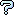All about flooble | fun stuff | Get a free chatterbox | Free JavaScript | Avatarsperplexus dot infoEqual Angles (Posted on 2005-12-14)Let circle A be in the interior of circle B and tangent to it at point M. Let chord QR of circle B be tangent to circle A at point P. Prove that angles PMQ and PMR are equal.

1993 British Mathematical Olympiad,Round 1,Problem 4.

 See The Solution Submitted by Bractals No RatingComments: ( Back to comment list | You must be logged in to post comments.)re: Is there an easier way? ???| Comment 2 of 4 |(In reply to Is there an easier way? by DrBob)

>Construct MN perpendicular to the tangent at M - this passes >through the centre of both circles. Call the centre of A, O

Why call the certre of A, O?  The center of A is A.

Where exactly is N?  Is MN a diameter of circle A?  If so that would make angle MPN = 90 but it would certainly not be true that angle NMR = angle NPR

 Posted by Jer on 2005-12-14 14:28:39Please log in:

 Search: Search body:
Forums (0)